# Comments on Simulating Moving Sound Sources

Here’s an extension of what is covered in the book:

Suppose you want to synthesize the sound field that is radiated by a monopole sound source that moves uniformly and parallel to the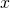-axis, but the secondary source distribution is not parallel to the-axis. Here’s the moving source’s sound field (That’s actually Fig. 5.45(a) in the book):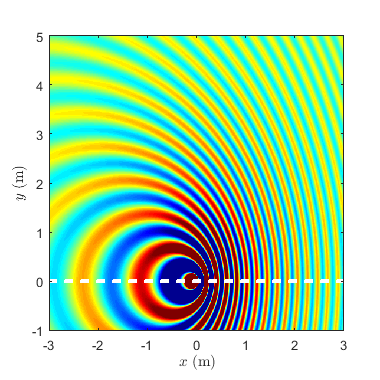The white dashed line represents the source’s trajectory.

Below is the result for an angled secondary source distribution. Actually, all equations that are necessary for the implementation are contained in the book. Check the Matlab script to see how I did it (remove the .txt file name extension before running it).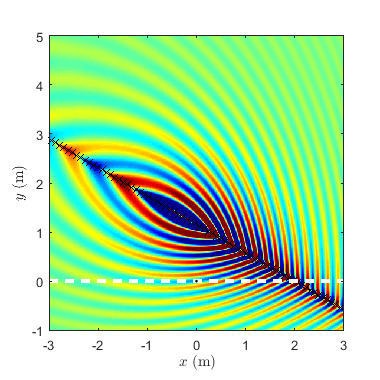Now, suppose you want to synthesize the sound field that is radiated by a monopole sound source that moves along a trajectory that is not parallel to the-axis. The sound field of such a source might look like this: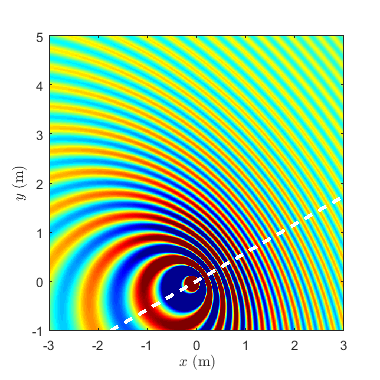The trick is rotating the coordinate system and using the equations in the book. The Matlab script is here.

And finally, above sound source synthesized using WFS: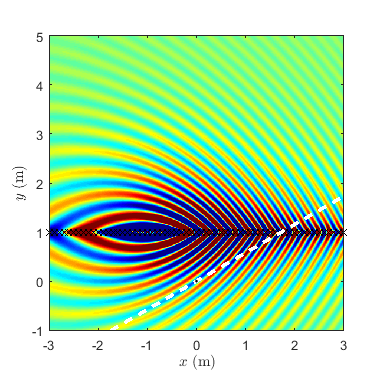The Matlab script is here. You can, of course, rotate the source trajectory and the secondary source distribution at the same time. I’ll leave it to you to do that.